# Unit IV: Electromagnetic Induction And Alternating Current - Online Test

Q1. An electron moves on a straight line path XY as shown in the figure. The coil abcd is adjacent to the path of the electron. What will be the direction of current, if any, induced in the coil?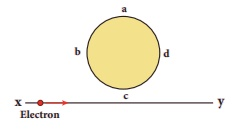Explaination / Solution: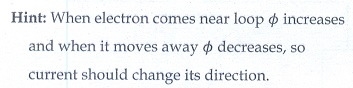Q2.

A thin semi-circular conducting ring (PQR) of radius r is falling with its plane vertical in a horizontal magnetic field B, as shown in the figure.The potential difference developed across the ring when its speed v , is
Explaination / Solution: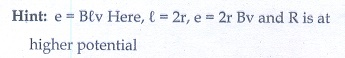Q3. The flux linked with a coil at any instant t is given by ΦB = 10t 2 50t + 250. The induced emf at t = 3s is
Explaination / Solution:Q4. When the current changes from +2A to 2A in 0.05 s, an emf of 8 V is induced in a coil. The co-efficient of self-induction of the coil is
Explaination / Solution:Q5. The current i flowing in a coil varies with time as shown in the figure. The variation of induced emf with time would be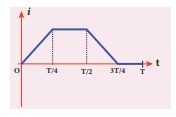Explaination / Solution: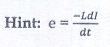Q6. A circular coil with a cross-sectional area of 4 cm2 has 10 turns. It is placed at the centre of a long solenoid that has 15 turns/cm and a cross-sectional area of 10 cm2. The axis of the coil coincides with the axis of the solenoid. What is their mutual inductance?
Explaination / Solution: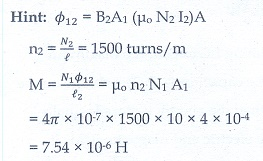Q7. In a transformer, the number of turns in the primary and the secondary are 410 and 1230 respectively. If the current in primary is 6A, then that in the secondary coil is
Explaination / Solution: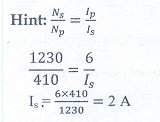Q8. A step-down transformer reduces the supply voltage from 220 V to 11 V and increase the current from 6 A to 100 A. Then its efficiency is
Explaination / Solution:Q9. In an electrical circuit, R, L, C and AC voltage source are all connected in series. When L is removed from the circuit, the phase difference between the voltage and current in the circuit is π /3 . Instead, if C is removed from the circuit, the phase difference is again π / 3 . The power factor of the circuit is
Explaination / Solution: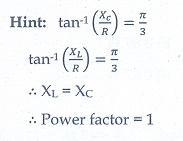Q10. In a series RL circuit, the resistance and inductive reactance are the same. Then the phase difference between the voltage and current in the circuit is
Explaination / Solution: